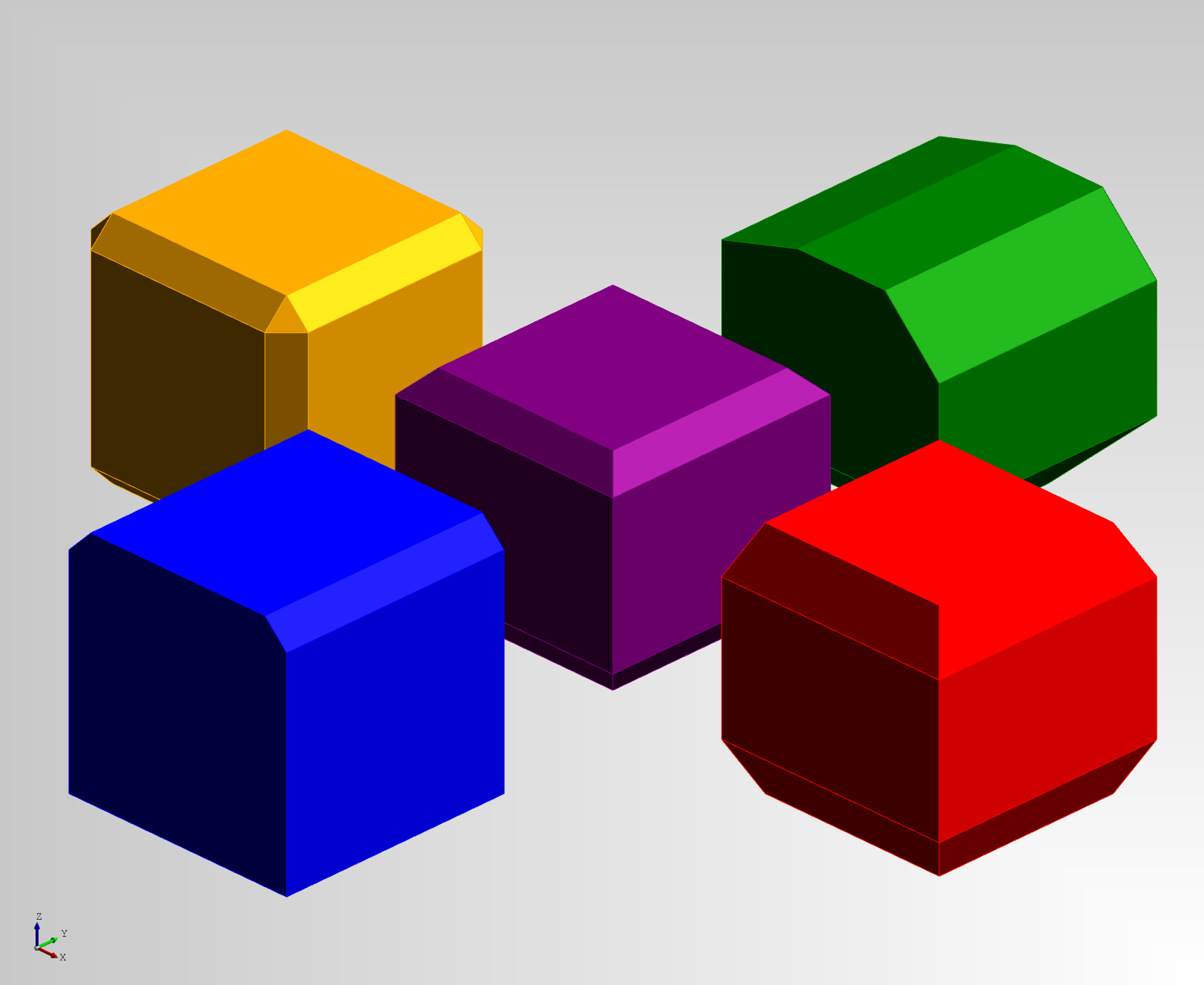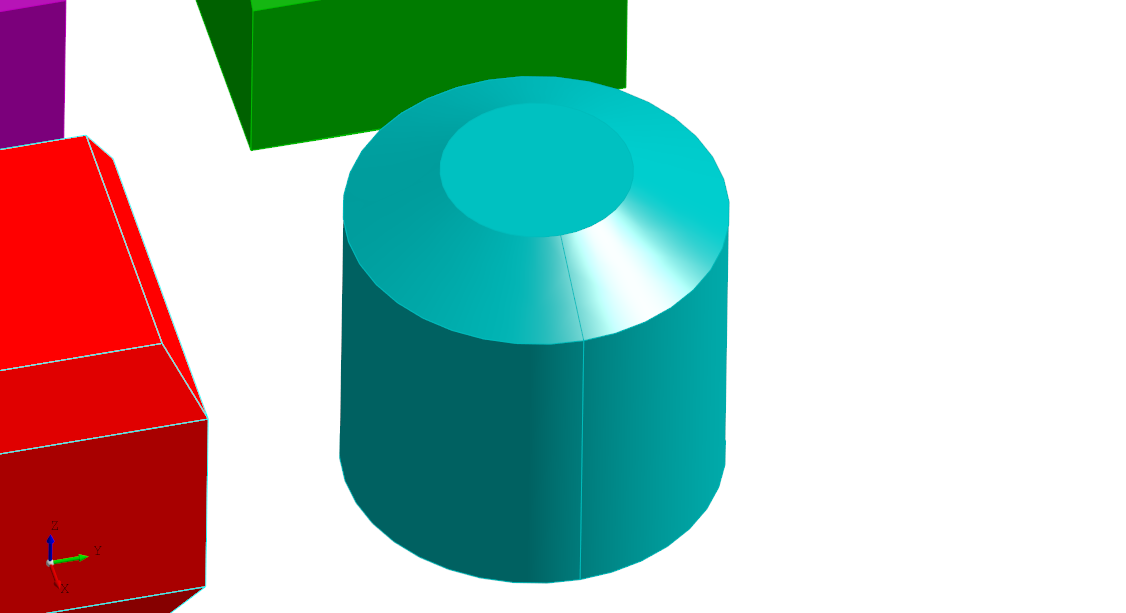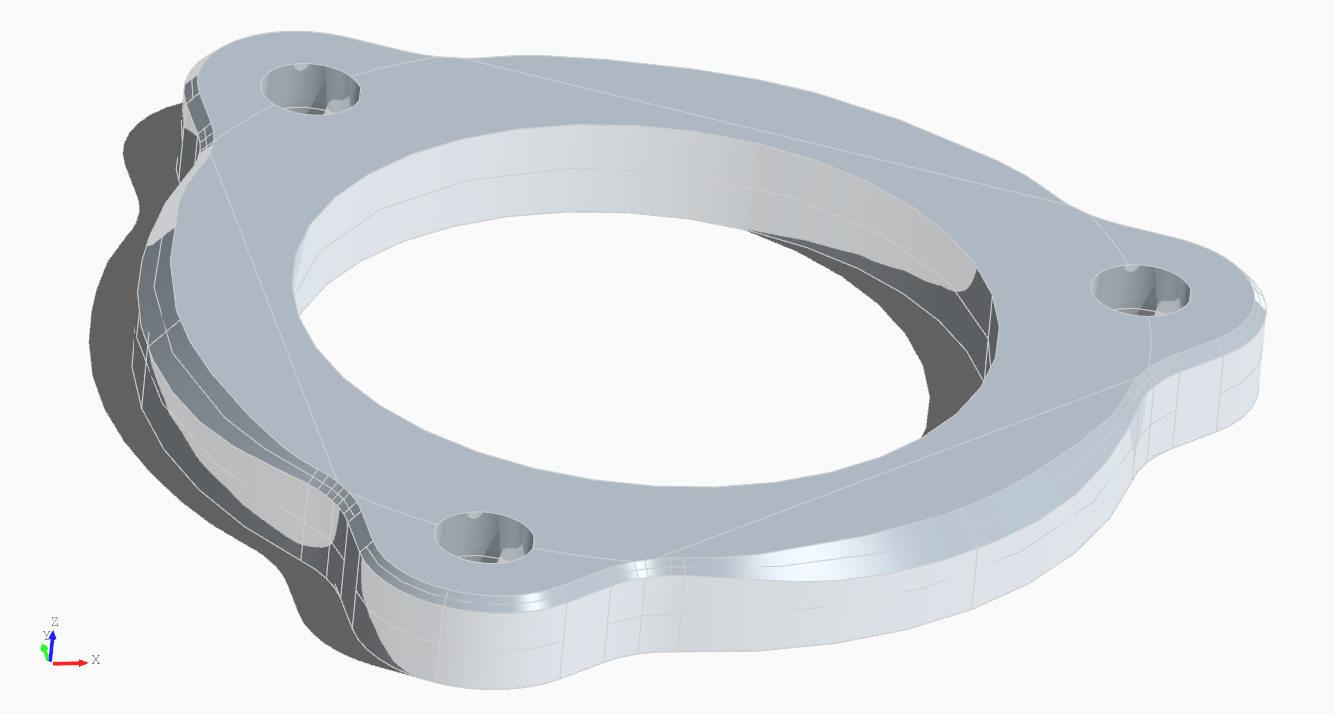## Chamfer

The `Chamfer` declaration can be used to add a straight cut to edges on a shape. There's several different types of chamfer operations that can be performed so let's cover each.The screenshot above demonstrates the following chamfer operations:

• Chamfering all edges of a shape with a the same `distance` and optional `distance2` (shown in orange)
• Chamfering only faces given in the operations list with the same `distance` and optional `distance2` attribute (shown in red)
• Chamfering specific sets of faces with specific dstances by defining them in the `operations` list (shown in purple)
• Chamfering specific sets of edges and faces pairs by defining them in the `operations` list (shown in blue)
• Chamfering any combination of the above by setting them in the in the `operations` list (shown in green)

The code for the chamfer example is as follows:

``````""" This example shows how to use chamfer operations

for more on how this works.

"""

from declaracad.occ.api import Part, Box, Chamfer

enamldef Assembly(Part):
name = "Chamfer Operations"

Chamfer:
# If no operations are given it applies to all faces
distance = 20
Box:
position = (-300, 0, 0)
dx = 200
dy = 200
dz = 200

Chamfer:
# Or you can supply which faces to apply the chamfer to
# and you can change the slope by specifying distance2
color = 'red'
distance = 20
distance2 = 40
attr top_face = self.children.topology.faces
attr bottom_face = self.children.topology.faces
operations = [top_face, bottom_face]
Box:
position = (300, 0, 0)
dx = 200
dy = 200
dz = 200

Chamfer:
# You also supply specify different chamfer distances for each chamfer
color = 'purple'
operations = [
(20, self.children.topology.faces),
(50, self.children.topology.faces),
]
Box:
dx = 200
dy = 200
dz = 200

Chamfer:
# You can supply a tuple of (edge, face) to define which edges of a
# given face should be chamfered
color = 'blue'
distance = 20
attr top_face = self.children.topology.faces
attr top_edges = self.children.topology.edges_from_face(top_face)
operations = [
(top_edges, top_face),
(top_edges, top_face)
]
Box:
position = (0, -300, 0)
dx = 200
dy = 200
dz = 200

Chamfer:
# You also supply the chamfer distances for these as well
color = 'green'
attr top_face = self.children.topology.faces
attr bottom_face = self.children.topology.faces
attr top_edges = self.children.topology.edges_from_face(top_face)
operations = [
(70, 20, top_edges, top_face),
(50, top_edges, top_face),
(50, bottom_face)
]
Box:
position = (0, 300, 0)
dx = 200
dy = 200
dz = 200
``````

### Distance and angle

The `operations` list can now accept a `ChamferData` object (available `from declaracad.occ.api import ChamferData`) which supports the specifying a `distance` and `angle` in radians along with the face and reference edge.

``````    Chamfer:
# Using ChamferData
color = 'teal'
operations = [
ChamferData(
distance=50,
edge=cyl.topology.edges,
face=cyl.topology.faces,
)
]
Cylinder: cyl:
position = (400, 400, 0)
height = 200
``````### Variable width chamfer

DeclaraCAD can now also perform a chamfer with variable widths. To use it provide a list of parametric values and widths in the `operations` list along with the edge.``````enamldef CylinderEdge(Part):
attr h = 4
Chamfer:
material = 'aluminium'
color = 'slategray'
attr edges = plate.topology.edges
attr profile_edges = (58, 130, 163)
attr top_edges = (46, 50, 54, 58, 71, 130, 137, 141, 145, 154, 158, 162, 163, 170, 174, 178, 182, 186)
attr profile = [
(0, 0.5),
(0.2, 1),
(0.5, 1.2),
(0.8, 1),
(1, 0.5),
]
operations = [
(profile if i in profile_edges else 0.5, edges[i])
for i in top_edges
]
Cut: plate:
Fillet:
attr edges = self.children.topology.edges
operations = [
edges[i] for i in ( 24, 26, 28, 33, 38, 42, 46, 50, 54)
]
Fuse:
Cylinder:
height = h
Extrude:
vector = (0, 0, h)
Face:
Polygon:
co unt = 3
Cylinder: # Center hole